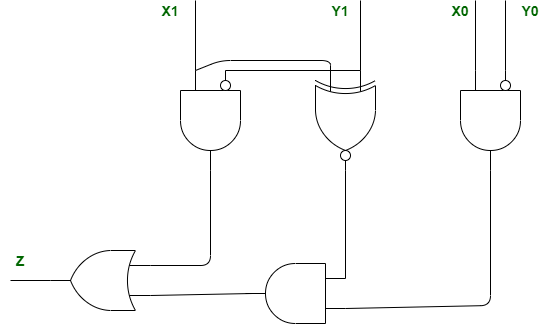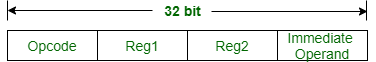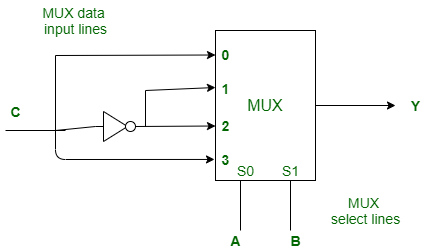# ISRO CS 2020

• Last Updated : 09 Aug, 2021

 Question 1
Regression testing is primarily related to
 A Functional testing B Development testing C Data flow testing D Maintenance testing
ISRO CS 2020
Discuss it

Question 1 Explanation:
Major Functional Testing Techniques:
• Unit Testing
• Integration Testing
• Smoke Testing
• User Acceptance Testing
• Interface Testing
• Usability Testing
• System Testing
• Regression Testing
So, option (A) is correct.
 Question 2
Of the following sort algorithms, which has execution time that is least dependent on initial ordering of the input ?
 A Insertion sort B Quick sort C Merge sort D Selection sort
ISRO CS 2020
Discuss it

Question 2 Explanation:
• Insertion Sort gives best case if initial input is already sorted.
• Quick Sort gives worst case if initial input is already sorted.
• Selection Sort gives least number of swaps if initial input is already sorted.
• Merge sort never depend on its initial order of input, does not matter what is input, it always takes O(n log n) time.
So, option (C) is correct.
 Question 3
The following circuit compares two 2-bit binary numbers, X and Y represented by X1X0 and Y1Y0 respectively. (X0 and Y0 represent Least Significant Bits)Under what condition Z will be 1 ?
 A X > Y B X < Y C X = Y D X! = Y
ISRO CS 2020
Discuss it

Question 3 Explanation:
Given, expression is,
`Z = X1Y1' + (X1⊙Y1)X0Y0'`
Therefore, if X > Y, then Z is 1. Option (A) is correct.
 Question 4
What is the availability of the software with following reliability figures.
```Mean Time Between Failures (MTBF) is 20 days
Mean Time To Repair (MTTR) is 20 hours ```
 A 90% B 96% C 24% D 50%
ISRO CS 2020
Discuss it

Question 4 Explanation:
Convert into the same unit then apply the formula. Software availability,
```= MTBF / (MTBF + MTTR)
= 20*24 / (20*24 + 20)
= 480 / 500
= 0.96
= 96 %```
Option (D) is correct.
 Question 5
What is the defect rate for Six sigma ?
 A 1.0 defect per million lines of code B 1.4 defects per million lines of code C 3.0 defects per million lines of code D 3.4 defects per million lines of code
ISRO CS 2020
Discuss it

Question 5 Explanation:
Six Sigma performance produces a defect-free product 99.99966% of the time; allowing only 3.4 errors per one million opportunities. 10 applications would need to be corrected during the entire year. Four sigma and six sigma levels of performance both have an error free rate over 99% of the time. Reference - Six Sigma. Option (D) is correct.
 Question 6
Consider a 5-segment pipeline with a clock cycle time 20 ns in each sub operation. Find out the approximate speed-up ratio between pipelined and non-pipelined system to execute 100 instructions. (If an average, every five cycles, a bubble due to data hazard has to be introduced in the pipeline.).
 A 5 B 4.03 C 4.81 D 4.17
ISRO CS 2020
Discuss it

Question 6 Explanation:
According to given data, speedup is
```= (Time taken by non-pipeline) / (Time taken by pipeline)
= (5*100*20) / {(100+20+5 - 1)*20}
= 4.03```
Option (B) is correct.
 Question 7
Consider a 32-bit processor which supports 70 instructions. Each instruction is 32 bit long and has 4 fields namely opcode, two register identifiers and an immediate operand of unsigned integer type. Maximum value of the immediate operand that can be supported by the processor is 8191. How many registers the processor has ?
 A 32 B 64 C 128 D 16
ISRO CS 2020
Discuss it

Question 7 Explanation:
Given, 32-bit processor,Number of bits required for Opcode = ceiling(log2(70)) = 7 Number of bits required for Immediate operands = ceiling(log2(8191)) = ceiling (12.99) = 13 Now, Number of bits left to represents register bits = 32 - (7+13) = 12. Since there are two register operands (i.e., Reg1 and Reg2), so 6 bits for each register. Hence, number of registers = 26 = 64 registers. Option (B) is correct.
 Question 8
In a 8-bit ripple carry adder using identical full adders, each full adder takes 34 ns for computing sum. If the time taken for 8-bit addition is 90 ns, find time taken by each full adder to find carry.
 A 6 ns B 7 ns C 10 ns D 8 ns
ISRO CS 2020
Discuss it

Question 8 Explanation:
Given,
```n = 8 Ripple Carry Adder
SUM delay from each Full Adder Sdelay = 34 ns
CARRY delay from each Full Adder Cdelay = ?
Overall delay = T = 90 ns ```
Now,
```T = Sdelay + (n – 1)Cdelay
90 = 34 + 7 × Cdelay
Cdelay = 8 ns ```
So, option (D) is correct.
 Question 9
Following Multiplexer circuit is equivalent toA Sum equation of full adder B Carry equation of full adder C Borrow equation for full subtractor D Difference equation of a full subtractor
ISRO CS 2020
Discuss it

Question 9 Explanation:
According to given Multiplexer,
```Y = A′B′C+A′BC′+AB′C′+ABC
Y = A⊕B⊕C
Y = Σm(1, 2, 4, 7)```
Which is equivalent to sum equation of full adder and also difference equation of a full subtractor. Both option (A) and (D) are correct.
 Question 10
A stack is implemented with an array of ‘A[0...N – 1]’ and a variable ‘pos’. The push and pop operations are defined by the following code.
```push (x)
A[pos] ← x
pos ← pos – 1
end push

pop ( )
pos ← pos + 1
return A[pos]
end pop ```
Which of the following will initialize an empty stack with capacity N for the above implementation ?
 A pos ← –1 B pos ← 0 C pos ← 1 D pos ← N – 1
ISRO CS 2020
Discuss it

Question 10 Explanation:
Since we are using an array as a stack, we have a choice between 0 or N-1. Looking at the code, Pop increment pos and push decrement pos. Stack is growing from larger index to lower index. Thus, for empty stack pos ← N – 1 is correct.
There are 80 questions to complete.
My Personal Notes arrow_drop_up